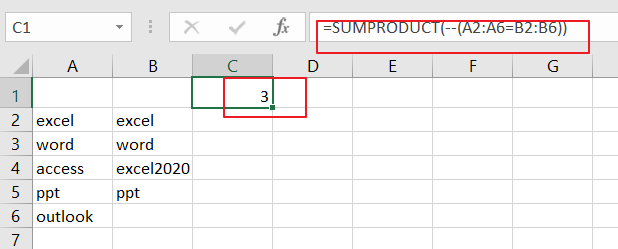# How to Count Matches between Two Columns in Excel

This post will guide you how to Count the number of matches between two columns using a formula in Excel 2013/2016 or Excel office 365. How do I compare two columns within two different ranges or columns and count if the value in column1 found in column2 in Excel. You can use the SUMPRODUCT function to compare two columns, and is used to multiply the corresponding components in the given two ranges or arrays and returns the sum of those products.

The Syntax of the SUMPRODUCS is as below:

=SUMPRODUCT(array1,[array2]…)

For Example, you have a data of list in both A2:A6 and B2:B6 and you wish to count any differences between those two ranges, and you can use the below SUMPRODUCT function:

=SUMPRODUCT(–(A2:A6=B2:B6))

###You can select cell C1 to place the final result, just click on it, and insert the above formula, then press Enter key.

### Related Functions

• Excel SUMPRODUCT function
The Excel SUMPRODUCT function multiplies corresponding components in the given one or more arrays or ranges, and returns the sum of those products. The syntax of the SUMPRODUCT function is as below:= SUMPRODUCT (array1,[array2],…)…

Sidebar